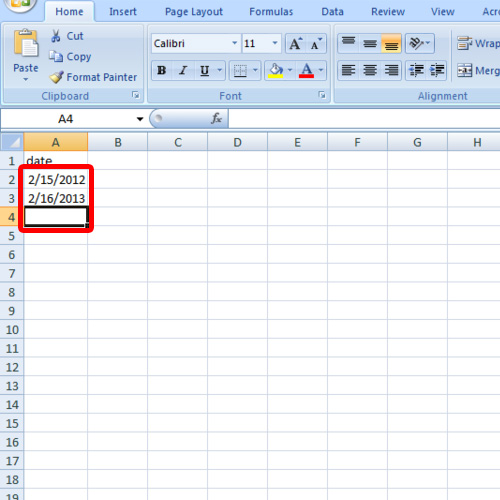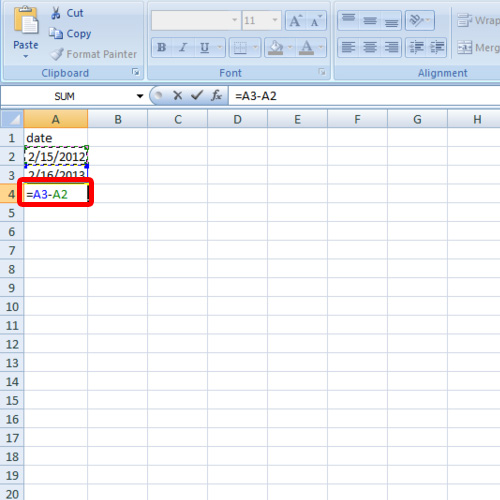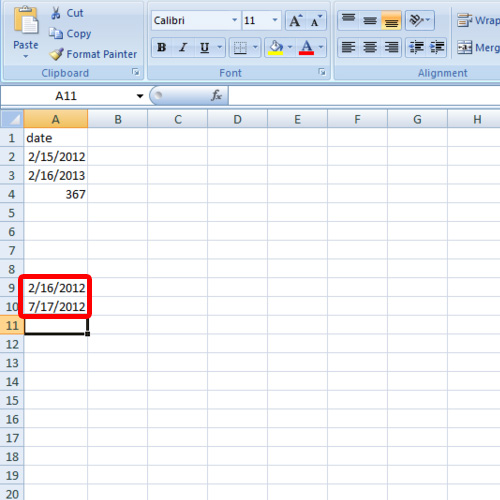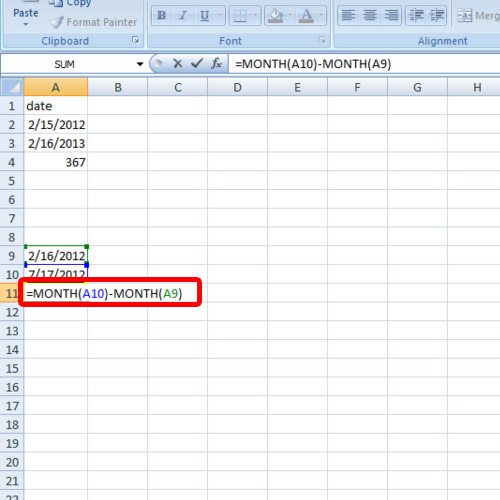## How to Calculate the Difference Between Two Dates

In this tutorial, we will teach you how to calculate the difference between two dates. We will also teach you how to calculate the difference in months between two dates.

Step 1 – Enter data

Follow this step by step guide to learn how to calculate the difference between two dates.

First of all, open an excel workbook. Enter the two dates between which you want to calculate the difference. For the purpose of this tutorial, we will enter the dates in the same column. The format in which the date is to be entered should be month/date/year. Once you have entered the two dates, go to the cell where you want to insert the formula.Step 2 – Difference between two dates

For the purpose of this tutorial, we will be calculating the difference between the dates entered in cells A3 and A2. Type =A3- A2. The difference between the two dates will be computed in days with the help of this formula.Step 3 – Enter data for monthly difference calculation

Next, we will show you how to find the month difference between two dates. In order to calculate this difference, you will have to type in two dates with different months.Step 4 – Month formula

For the purpose of this tutorial, we will be calculating the month difference between the cells A10 and A9. Now go to the cell where you want to place the formula and type the following:

=(Month(A10)-Month(A9))

Hit the enter key when you are done and the difference between the months of the two dates will be calculated.

In this manner, you can calculate the difference between two dates.